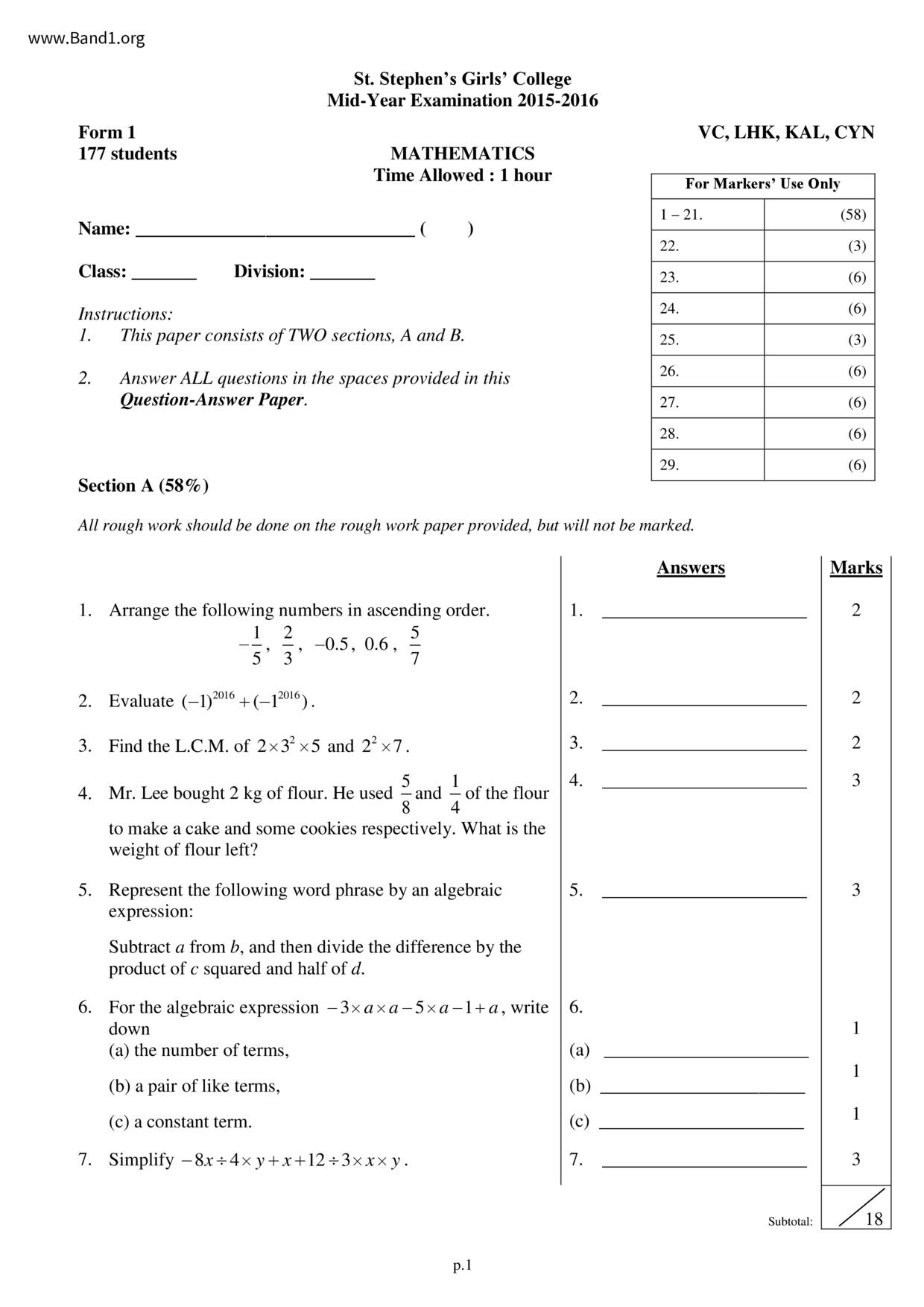# 中一 數學試卷 (F1 Maths Past Paper)

6932

pdf

8

st_stephen_girl_college_F1-Maths-15-16-my

▼ 圖片只作預覽, 如欲下載整份卷, 請按「免費成為會員」 ▼▲ 圖片只作預覽, 如欲下載整份卷, 請按「免費成為會員」 ▲

## 中一數學試卷 PDF 下載

177 students
Instructions:
St. Stephen's Girls' College
Mid-Year Examination 2015-2016
MATHEMATICS
Time Allowed : 1 hour
This paper consists of TWO sections, A and B.
Answer ALL questions in the spaces provided in this
1. Arrange the following numbers in ascending order.
2. Evaluate (-1) 2016 +(-12016).
3. Find the L.C.M. of 2x3²x5 and 2²x7.
4. Mr. Lee bought 2 kg of flour. He used
to make a cake and some cookies respectively. What is the
weight of flour left?
of the flour
5. Represent the following word phrase by an algebraic
expression:
Subtract a from b, and then divide the difference by the
product of c squared and half of d.
Section A (58%)
All rough work should be done on the rough work paper provided, but will not be marked.
6. For the algebraic expression - 3×a×a-5×a-1+a, write
(a) the number of terms,
(b) a pair of like terms,
(c) a constant term.
7. Simplify - 8x÷4×y+x+12÷3xxxy.
VC, LHK, KAL, CYN
For Markers' Use Only
F.1 Maths. Mid-year Exam. 2015-2016
8. Mary has p dozen pencils. After she gives 7 pencils to her
brother, how many pencils does she have? Express your
9. Solve the following equations:
(a) x 24 = 2x
(b) -5x=11-2(4x-5)
10. It is given that y=-2-x². Find the value of y when
11. It is given that -4,-2 and 0 are the solutions of an
inequality. Which of the following could be the inequality?
2x+4<-4, 2x+4≤-4, 2x+4>-4, 2x+4≥ −4
12. Which of the following angle has the 2nd greatest value? 12.
of a round angle,
of a straight angle,
of a right angle.
13. Find ZEDF in the following diagram.
14. What percentage of 6 m is 54 cm?
15. If 15% of y is 12, find the value of y.
16. The number of students in a school increases from 750 to 16.
800. Find the percentage increase.
17. As compared to last month, the price of a model car 17.
increases by 36% this month. the price of the model car
is \$340 this month, what was the price of the model car last
18. Betty spent \$1 200 on food and \$800 on transportation last 18.
month. She spends 16% less on food and 12% more on
transportation this month. What is the percentage change in
her total expenditure on food and transportation?
F.1 Maths. Mid-year Exam. 2015-2016
19. A dress marked at \$450 is sold at a 20% discount. If the 19.
percentage profit is 12.5%, find the cost price of the dress.
20. Draw the 2-D representation of the solid below on the
given isometric grid paper.
21. Find the total number of small squares and the number of 21.
white small squares in the 25th figure.
22. Evaluate-5--
Section B (42%) All working must be clearly shown in the spaces provided.
Total number
of small squares =
white small squares =
F.1 Maths. Mid-year Exam. 2015-2016
23. Tom and Kevin took part in a competition. 2 marks were awarded for each correct answer and
1 mark was deducted for each wrong answer. Tom answered 12 questions and got 9 correct
(a) Find Tom's score.
(b) Kevin answered 10 questions. His score was 5 marks higher than Tom's. Find the number of
24. A boat sailed along a river 1.5 km/h to the east and 2 km/h to the west. Suppose +1 km'
represents that the boat is 1 km to the east of the starting point.
(a) If the boat spent 4 hours travelling due east and then 3 hours travelling due west, what is the
final position of the boat relative to the starting point?
(b) Suppose the boat travelled for 5 hours from the starting point. During the journey, it spent
x hours travelling due east and the rest of the time due west. Let d km be the final position of
the boat relative to the starting point.
(i) Express d in terms of x.
(ii) Write down two possible values of x such that the boat is to the west of the starting point
after the whole journey.
F.1 Maths. Mid-year Exam. 2015-2016
25. The general term of a sequence is
26. (a) Use a pair of compasses and a ruler to construct APQR where PQ = 4 cm and
PR = QR = 3 cm in the space below.
(a) and (c)
(b) Measure and write down the value of ZPRQ.
(c) Extend QR to a point N where RN = QR. Join NP.
(d) (i) According to the lengths of the sides of the triangle, what kind of triangle is APNR?
(ii) According to the sizes of the angles in the triangle, what kind of triangle is APNQ?
(d) (i) APNR is
(ii) APNQ is
F.1 Maths. Mid-year Exam. 2015-2016
27. Solve the equation
1 [2x - (x + 1)] = 5-1/(x-2).
F.1 Maths. Mid-year Exam. 2015-2016
28. In a bag, there are 48 balls, where 16% are green and the rest are white.
Find the number of white balls in the bag.
If Alice puts some white balls into the bag, is it possible that exactly 85% of the balls in the